Noel, Jonathan A.

Weak saturation of the cube in the clique ★

Author(s): Morrison; Noel

Problem

Determine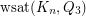.

Extremal $4$-Neighbour Bootstrap Percolation in the Hypercube ★★

Author(s): Morrison; Noel

Problem   Determine the smallest percolating set for the-neighbour bootstrap process in the hypercube.

Saturation in the Hypercube ★★

Author(s): Morrison; Noel; Scott

Question   What is the saturation number of cycles of lengthin the-dimensional hypercube?

Keywords: cycles; hypercube; minimum saturation; saturation

Cycles in Graphs of Large Chromatic Number ★★

Author(s): Brewster; McGuinness; Moore; Noel

Conjecture   If, thencontains at least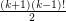cycles of length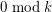.

Keywords: chromatic number; cycles

Saturated $k$-Sperner Systems of Minimum Size ★★

Author(s): Morrison; Noel; Scott

Question   Does there exist a constantand a functionsuch that if, then every saturated-Sperner systemhas cardinality at least?

Partitioning the Projective Plane ★★

Author(s): Noel

Throughout this post, by projective plane we mean the set of all lines through the origin in.

Definition   Say that a subsetof the projective plane is octahedral if all lines inpass through the closure of two opposite faces of a regular octahedron centered at the origin.
Definition   Say that a subsetof the projective plane is weakly octahedral if every setsuch thatis octahedral.
Conjecture   Suppose that the projective plane can be partitioned into four sets, say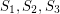andsuch that each setis weakly octahedral. Then eachis octahedral.

Keywords: Partitioning; projective plane

Choosability of Graph Powers ★★

Author(s): Noel

Question  (Noel, 2013)   Does there exist a function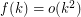such that for every graph,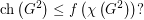Choice Number of k-Chromatic Graphs of Bounded Order ★★

Author(s): Noel

Conjecture   Ifis a-chromatic graph on at mostvertices, then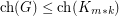.

Mixing Circular Colourings ★

Author(s): Brewster; Noel

Question   Is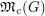always rational?

Keywords: discrete homotopy; graph colourings; mixing